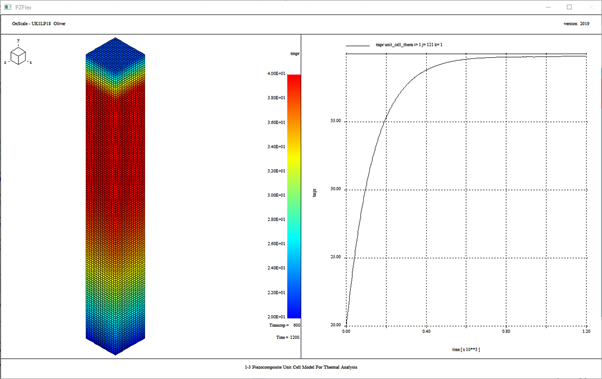# Thermal Analysis Unit Cell

## Model Description

1-3 Piezocomposite unit cell model can be used evaluate the fundamental microstructure of your design.

This example shows how to calculate the internal heating in a composite pillar due to losses in the constituent materials. The unit cell (figure 1) is fired in a pulsed mode meaning the method for calculating thermal response is slightly different to that of a continuous wave (cw) excitation. With pulsed excitation a short broadband pulse of ultrasound is transmitted, and the model is run until all significant waves have left the model or have been absorbed at the boundaries. Data is gathered over the entire simulation for loss (energy deposition)Figure 1. Material Model

The thermal example shows how to determine heat losses created in a piezoelectric model by splitting the mechanical and thermal simulations into two sections. The thermal example shows how to determine heat losses created in a piezoelectric model by splitting the mechanical and thermal simulations into two sections. First you model the acoustic wave interactions in the model and then you use the resulting acoustic loss data to model the thermal source in the pillar. The decoupling of the two models allows for efficient modelling, as they occur on vastly different time scales.

## Typical Outputs from Thermal Analysis

• Temperature rise
• Displacement
• Thermal stress

## Runtime Statistics

 Model size 14208 elements Solve Time 19 s (2 CPU) Core Hours 0.011 Memory Usage 170 MB Recommended Cloud Node Setting 2 CPU